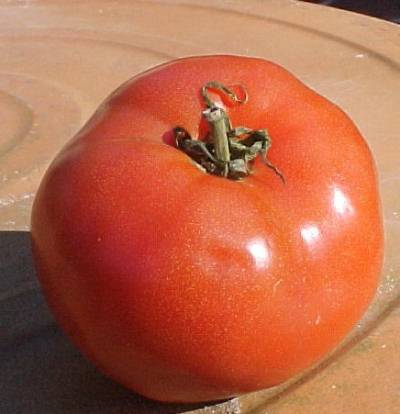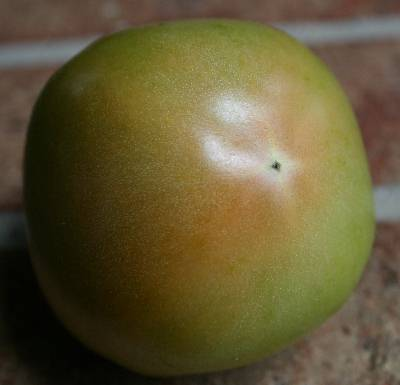# Tomato – How Long to Ripen (Extending Summer Season)Q: I would like to have ripe tomatoes throughout the summerAre there early, mid and late season varieties?

A: Yes – there are varieties that claim to ripen earlier or later than others but predicting exactly when a tomato will ripen is a very inexact science.

Tomatoes ripen in response to air temperature, day length, nutrient availability and a host of other factors.

Temperature seems to be the most important, so plant scientists have studied how many days, at temperatures above a certain threshold, it takes a tomato to ripen.

The unit scientists use is Growing Degree Days (GDD). A Growing Degree day is calculated by adding the low temperature of a day to the high temperature of the day and dividing by 2. From the result, 50 is subtracted…because most plants don’t grow at temperatures below 50 degrees.

Example: High temp = 92, low temp = 68
92 + 68 = 160
160/2 = 80
80 – 50 = 30 GDD

Example: High temp = 68, low temp = 42
68 + 42 = 110
110/2 = 55
55 – 50 = 5 GDD

Example: High temp = 66, low temp = 34
66 + 34 = 100
100/2 = 50
50 – 50 = 0 GDD

Tomatoes need somewhere above 1300 GDD to ripen. But remember that ripening is affected by MANY other factors. Some varieties ripen with less GDD…but gardeners report these tomatoes do not taste as good as tomatoes exposed to more heat.

You can get the number of GDD for your area by going to Georgia Weather and click on Calculators.